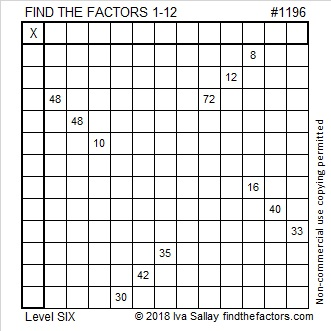# 1196 and Level 6

In this puzzle, the permissible common factors of 48 and 72 are 6, 8, and 12. For clues 8 and 16, you can choose from common factors 2, 4, or 8. Which choices will make the puzzle work? I’m not telling, but I promise that the entire puzzle can be solved using logic and a basic knowledge of a 12×12 multiplication table. There is only one solution.Print the puzzles or type the solution in this excel file: 12 factors 1187-1198

Here are some facts about the number 1196:

• 1196 is a composite number.
• Prime factorization: 1196 = 2 × 2 × 13 × 23, which can be written 1196 = 2² × 13 × 23
• The exponents in the prime factorization are 2, 1, and 1. Adding one to each and multiplying we get (2 + 1)(1 + 1)(1 + 1) = 3 × 2 × 2 = 12. Therefore 1196 has exactly 12 factors.
• Factors of 1196: 1, 2, 4, 13, 23, 26, 46, 52, 92, 299, 598, 1196
• Factor pairs: 1196 = 1 × 1196, 2 × 598, 4 × 299, 13 × 92, 23 × 52, or 26 × 46
• Taking the factor pair with the largest square number factor, we get √1196 = (√4)(√299) = 2√299 ≈ 34.583231196 is the hypotenuse of a Pythagorean triple:
460-1104-1196 which is (5-12-13) times 92

1196 is a palindrome in three different bases:
It’s 14241 in BASE 5,
838 in BASE 12, and
616 in BASE 14